Courses

# Test: Magnetism (Competition Level 1)

## 30 Questions MCQ Test Physics Class 12 | Test: Magnetism (Competition Level 1)

Description
This mock test of Test: Magnetism (Competition Level 1) for Class 12 helps you for every Class 12 entrance exam. This contains 30 Multiple Choice Questions for Class 12 Test: Magnetism (Competition Level 1) (mcq) to study with solutions a complete question bank. The solved questions answers in this Test: Magnetism (Competition Level 1) quiz give you a good mix of easy questions and tough questions. Class 12 students definitely take this Test: Magnetism (Competition Level 1) exercise for a better result in the exam. You can find other Test: Magnetism (Competition Level 1) extra questions, long questions & short questions for Class 12 on EduRev as well by searching above.
QUESTION: 1

### A point charge is moving in a circle with constant speed. Consider the magnetic field produced by the charge at a fixed point P (not centre of the circle) on the axis of the circle.

Solution:

(a) The point charge moves in circle as shown in Fig.
The magnetic field vectors at a point P on the axis of circle
are B→AandB→CB→AandB→C at the instant the point charge is at A and C
respectively, as shown in Fig.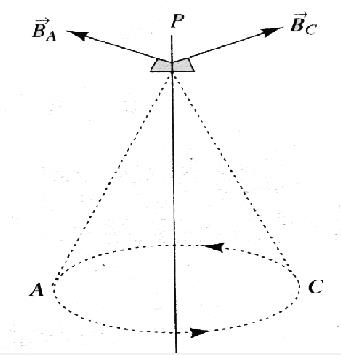QUESTION: 2

### Four infinitely long `L' shaped wires, each carrying a current i have been arranged as shown in the figure. Obtain the magnetic field intensity at the point `O' equidistant from all the four corners.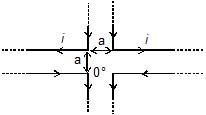Solution:

We know that,

B = μoI/4πa(sinθ2+sinθ1)

The net magnetic field due to wires from left to night and from right to left is zero as they cancel out. By applying the right-hand thumb rule. As the left sides, the magnetic field adds up & direction is upward whereas the right-side magnetic field adds up to but direction is downward. So, cancels up.

And for the wires from up to down or down to up, it is the wires cancel its conjugate according to right-hand thumb-rule.

Thus, net magnetic field at a point is 0.

QUESTION: 3

### Two parallel, long wires carry currents i1 and i2 with i1 > i2. When the current are in the same direction, the magnetic field at a point midway between the wire is 10 mT. If the direction of i2 is reversed, the field becomes 30mT. The ratio i1/i2 is

Solution: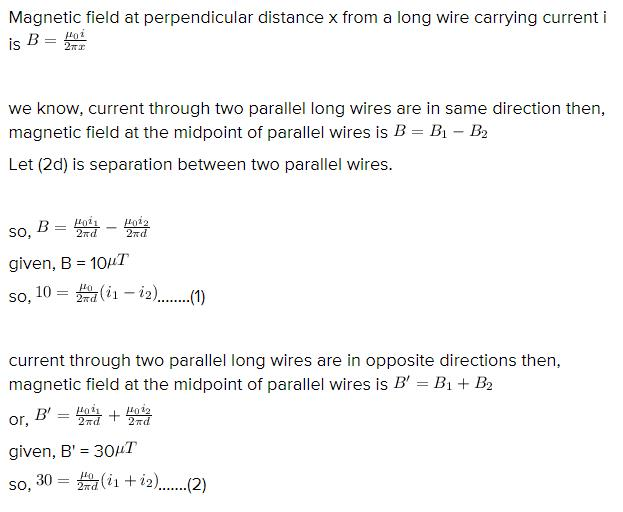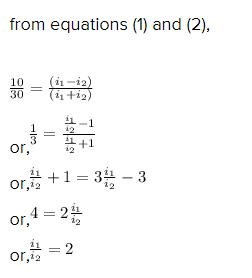QUESTION: 4

Two mutually perpendicular insulated long conducting wires carrying equal currents I, intersect at origin. Then the resultant magnetic induction at point P (2m, 3m) will be -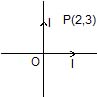Solution: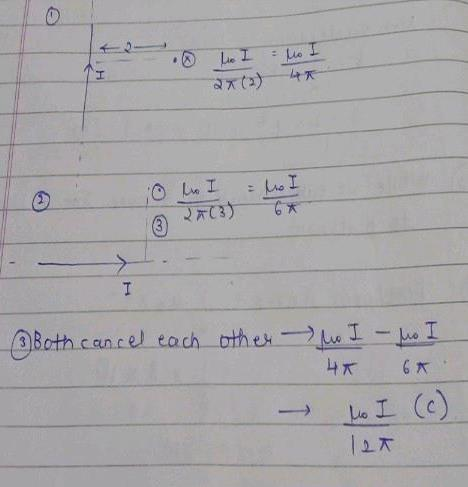QUESTION: 5

Two parallel straight long conducting wires, which are placed at a distance r from each other, are carrying equal currents i in opposite directions. The value of magnetic induction at a point situated at a point situated x from one wire in between the wires will be

Solution: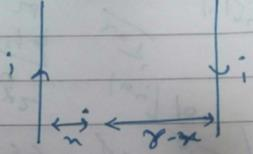Force due to both wires are inward.
=2 x (μo​I/4π)[(1/(r-x))+(1/x)]
=(μo​I/2π)[(1/(r-x))+(1/x)]

QUESTION: 6

Two very long straight parallel wires, parallel to y-axis, carry currents 4I and I, along +y direction and _ y direction respectively. The wires are passes through the x-axis at the points (d, 0, 0) and (-d, 0, 0) respectively. The graph of magnetic field z-component as one moves along the x-axis from x = _ d to x = +d, is best given by

Solution: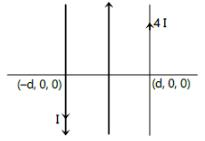Magnetic field at some x is given by,
0(4I)/2π(d-x)]k+ [μ0I/2π(d+x)]k
= μ0/2π[(4/d-x)+(1/d+x)]
= μ0/2π[5d+3x/d2-x2]
The correct answer is option C.

QUESTION: 7

If the ratio of magnetic fields at two point in a definite direction due to a long current carrying wire is 3/4, then the ratio of the distances of these points from the wire will be :

Solution:

B1= μ0ni/2r1
B2= μ0ni/2r2
B1/B2=r2/r1=3/4
r1:r2=4/3

QUESTION: 8

The points A and B are situated perpendicular to the axis of 2 cm long bar magnet at large distances x and 3 x from the centre on opposite sides. The ratio of magnetic fields at A and B will be approximately equal to

Solution:

Magnetic field at the axial of bar magnet is given by the relation as :
B=(μO/2π)​​(M​/d3)
where d is the distance
So,
B∝1/d3​
​BA/BB ​​= dB3​/ dA3
​BA/ BB​​=27/1​
Hence, the ratio of magnetic field of A and B is 27:1.

QUESTION: 9

Magnetic lines of force are

Solution:

Magnetic lines of force are continuous.
The tangent at each point of the magnetic field lines the direction of the magnetic field. And we know that magnetic fields exist in each and every point  in the vicinity of the magnet.

QUESTION: 10

Magnetic monopoles cannot exist

Solution:
QUESTION: 11

A battery is connected between two points A and B the circumference of a uniform conducting ring of radius r and resistance R. One o the arcs AB of the ring subtends an angle q at the centre. The value of the magnetic induction at the centre due to the current in the ring is

Solution:

Magnetic field at the centre due to current ABC arc is
B1​= (μ0​​/4π)(2πI1/r)​​(q/2π)    (Directed upwards)
Magnetic field at the centre due to current in ADC arc is
B2​=μ0​​2πI2​​(2π−q)/4π r 2π     (Directed downwards)
Therefore, net magnetic field at the centre
B=[μ0​​2πI1​​q/ 4πr2π] −[2πI2​​(2π−q) /r​ 2π]
Also, I1​= V/R1​ ​=[V/(ρl1​/A)]​= VA/ρrq​ and
I2​= V/R2​ ​= E/(ρl2​/A) ​= VA​/ρr(2π−q)
∴B= (μ0/4π​​)[(VA/ρrq)​×(q/r)​− (VA /ρr(2r−q)) ​×((2π−q)/r)​]=0

QUESTION: 12

Two circular coils of wire each having a radius of 4 cm and 10 turns have a common axis and are 6 cm apart. If a current of 1 A passess through each coil in the opposite direction find the magnetic induction. At a point on the axis, midway between them.

Solution:

The magnetic field due to a current carrying coil at the axis of the coil at a distance x from the centre of coil having N turns is given by,
B= μ0NIr2/2(r2+x2)3/2
So, here I=1A, N=10, r=4x10-2m and x=6x10-2m
B1=2π x 10 x 1 x (16x10-4/16+36)3/2 x-10-6)
B1=2.67x10-5T=0.264x10-4T
B=μ0NI/2r so,
B2=[(2πx10-7x10x1)/(2x4x10-2)]T
B2=1.57x10-4T
And since the current in both coil are same but in the in the opposite direction so magnetic fields at centre of either coils would be opposite so net magnetic field at the centre of either coil is,
B=B2-B1
B=(1.57-0.267)x10-4T
B=1.303x10-4T
The magnetic field at the midway of the two centres would be zero because the magnetic field at this point due to both coil is equal but opposite due to opposite current flowing in the coils. thus, B=0.
Hence  option B is correct.

QUESTION: 13

A direct current is passing through a wire. It is bent to form a coil of one turn. Now it is further bent to form a coil of two turns but at smaller radius. The ratio of the magnetic induction at the centre of this coil and at the centre of the coil of two turn is

Solution:
QUESTION: 14

If a copper rod carries a direct current, the magnetic field associated with the current will be [CPMT 1984]

Solution:
QUESTION: 15

Two wire loops PQRSP formed by joining two semicircular wires of radii R1 and R2 carries a current I as shown in (fig.) The magnitude of the magnetic induction at the centre C is ........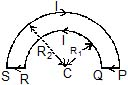Solution:

Magnetic field at the centre of a coil  Bc​=μo​IN​/2r
where N is the number of turns.
For semi-circular coil N=0.5
So, magnetic field at C due to semi-circular coil of radius R1​,
B1​=​ μo​I(0.5)/2R1​=​μo​I​/4R1  out of plane of paper
So, magnetic field at C due to semi-circular coil of radius R2​,
B2​=​μo​I(0.5)/2R2​=​μo​I​/4R2  into the plane of paper
Magnetic field at C due to section SR and PQ is zero.
So net magnetic field at C   Bnet​=B1​−B2​=(μo​I/4)​[(1/R1)​​−(1/R2​)​]

QUESTION: 16

In a coaxial, straight cable, the central conductor and the outer conductor carry equal currents in opposite directions. The magnetic field is zero.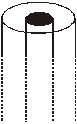Solution:

B=Bouter+Binner
Since the current carried by the outer and inner are equal and in opposite direction,
magnetic field produced by the cylinders at a point P (distance r from central axis) is
Bouter= (μ0/4π)i(dl×r/r3)
Binner=−(μ0/4π)i(dl×r/r3)
∴B=Bouter+Binner=0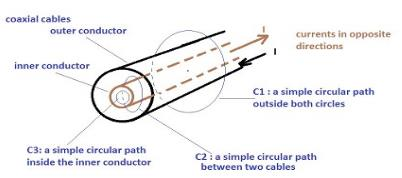QUESTION: 17

The magnetic field B inside a long solenoid, carrying a current of 10 A, is 3.14 × 10-2 T. Find the number of turns per unit length of the solenoid.

Solution: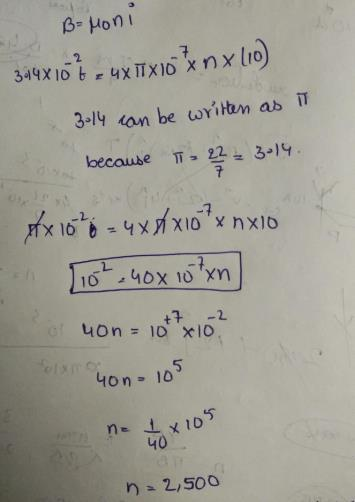QUESTION: 18

A rod AB moves with a uniform velocity v in a uniform magnetic field as shown in figure.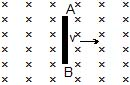Solution:

Force on positive charges in the rod will be in direction given by qv×B, i.e., towards A.
Hence force on the electron will be in the opposite direction i.e., towards B. So the negative charge will move towards B.
Therefore, B will be negatively charged and A will be positively charged.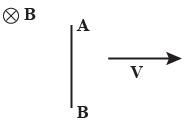QUESTION: 19

A charged particle is accelerated through a potential difference of 12 kV and acquires a speed of 1.0 × 106 m/s. It is then injected perpendicularly into a magnetic field of strength 0.2 T. Find the radius of the circle described by it.

Solution:

F(m) = qvB sin(90°)
Applied potential difference "V" = 12 kV = 12 × 103 V
Speed of a charged particle, v =1.0 × 106 m s−1
Magnetic field strength "B" = 0.2 T
As per the question, a charged particle is injected perpendicularly into the magnetic field.
We know:
qV = (½)mv2
=>m / q = (½)mv2
= (2 × 12×103)/ (1×106)2
= 24×10−9
r = mv / qB
r=(24×10−9 × 106) / 0.2
r = 12 × 10−2 m
= 12 cm
Thus, the radius of the circle is 12 cm.

QUESTION: 20

A neutron, a proton, an electron and an a-particle enters a uniform magnetic field with equal velocities. The field is directed along the inward normal to the plane of the paper. Which of these tracks followed are by a - particle.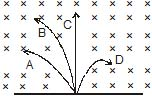Solution:

Path C is undeviated. Therefore, it is of neutron's path. From Fleming's left-hand rule magnitude force on positive charge will be leftwards and on negative charge is rightwards. Therefore, tack D is of electron. Among A and B one of proton and other of α−particle ≤.
Further, r=mv/Bq or r∝m/q
Since, (m/q)>(m/q)P
∴rα>rP
or track B is of α particle.

QUESTION: 21

A proton of mass 1.67 × 10-27 kg and charge 1.6 × 10-19C is projected with a speed of 2 × 106 m/s at an angle of 60° to the x-axis. If a uniform magnetic field of 0.104 T is applied along the y-axis, the path of te proton is

Solution:

vx​=vcosθ
vy​=vsinθ
Since vy​≠0, this component will be responsible for motion along the direction B( i.e y−axis).
The path will be helical and pitch will be constant.
Radius r of the circle perpendicular to the magnetic field B
r=mvcosθ/qB​=1.6×10−27×(2×106×cos600)/ 1.6×10−19×0.1​=0.1m
T=2πr/v​=2×π×0.1/2×106×cos600 ​=2π×10−7secs

QUESTION: 22

Three ions H+, He+ and O+2 having same kinetic energy pass through a region in which there is a uniform magnetic field perpendicular to their velocity, then

Solution:

Radius    r= √2mK​​/qB
⟹ r∝ √m/q​​
Because the deflection is inversely proportional radius so,
q/√m​=1/1​ : 1/√4 ​​: 2/√16​=1:1/2​:1/2​
Therefore, H+ deflects most and He+ and O+2 will be equally deflected.

QUESTION: 23

A permeable substance is one

Solution:

A permeable substance is one through which the magnetic lines of force can pass through easily.

QUESTION: 24

Electrons moving with different speeds enter a uniform magnetic field in a direction perpendicular to the field. They will move along circular paths.

Solution:

mv = BQr
or r = (m/BQ) v
As m, Q and B are the same for all the electrons, r ∝ v.

QUESTION: 25

In the previous question, time periods of rotation will be

Solution:
QUESTION: 26

A particle having charge of 1 C, mass 1 kg and speed 1 m/s enters a uniform magnetic field, having magnetic induction of 1 T, at an angle q = 30° between velocity vector and magnetic induction. The pitch of its helical path is (in meters)

Solution:

Pitch =Vcosθ×T
=Vcosθ×(2πm/qB)
Where m=1kg, q=1C, B=1T, V=1 m/s
=√3π .

QUESTION: 27

The direction of magnetic force on the electron as shown in the diagram is along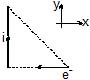Solution:

Magnetic field is in -Kdirection so direction of force.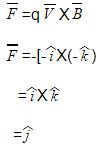So the answer is y axis.

QUESTION: 28

A particle moves in a region having a uniform magnetic field and a parallel, uniform electric field. at some instant, the velocity of the particle is perpendicular to the field direction. The path of the particle will be

Solution:

A charged particle moves in a circle when its velocity is perpendicular to the magnetic field. When it forms an acute angle with the magnetic field, it can be resolved in two components, parallel and perpendicular. The perpendicular components tends to move it in circle, the parallel components tends it to move along the magnetic field to form a helical motion of uniform radius and pitch.

QUESTION: 29

An electron is moving along positive x-axis. A uniform electric field exists towards negative y-axis. What should be the direction of magnetic field of suitable magnitude so that net force of electron is zero.

Solution:

Let the velocity of the electron is v

Then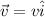If the electric field is E then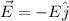Lorentz Force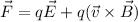or,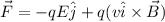For force to be zero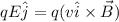or,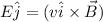To get the RHS in the direction of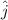, B should be acting in the direction of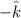. As we know that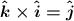Therefore the direction of the magnetic field must be in the negative z-axis.

QUESTION: 30

A charged particle is released from rest in a region of uniform electric and magnetic fields, which are parallel to each other. The locus of the particle will be

Solution:

When the particle is released from rest, it starts to move along the fields due to application of electric field.
The magnetic force acting on a moving charged particle is given by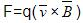Since velocity and magnetic fields are parallel, no magnetic force acts on the particle, hence it continues to move in a straight line along with constant acceleration.